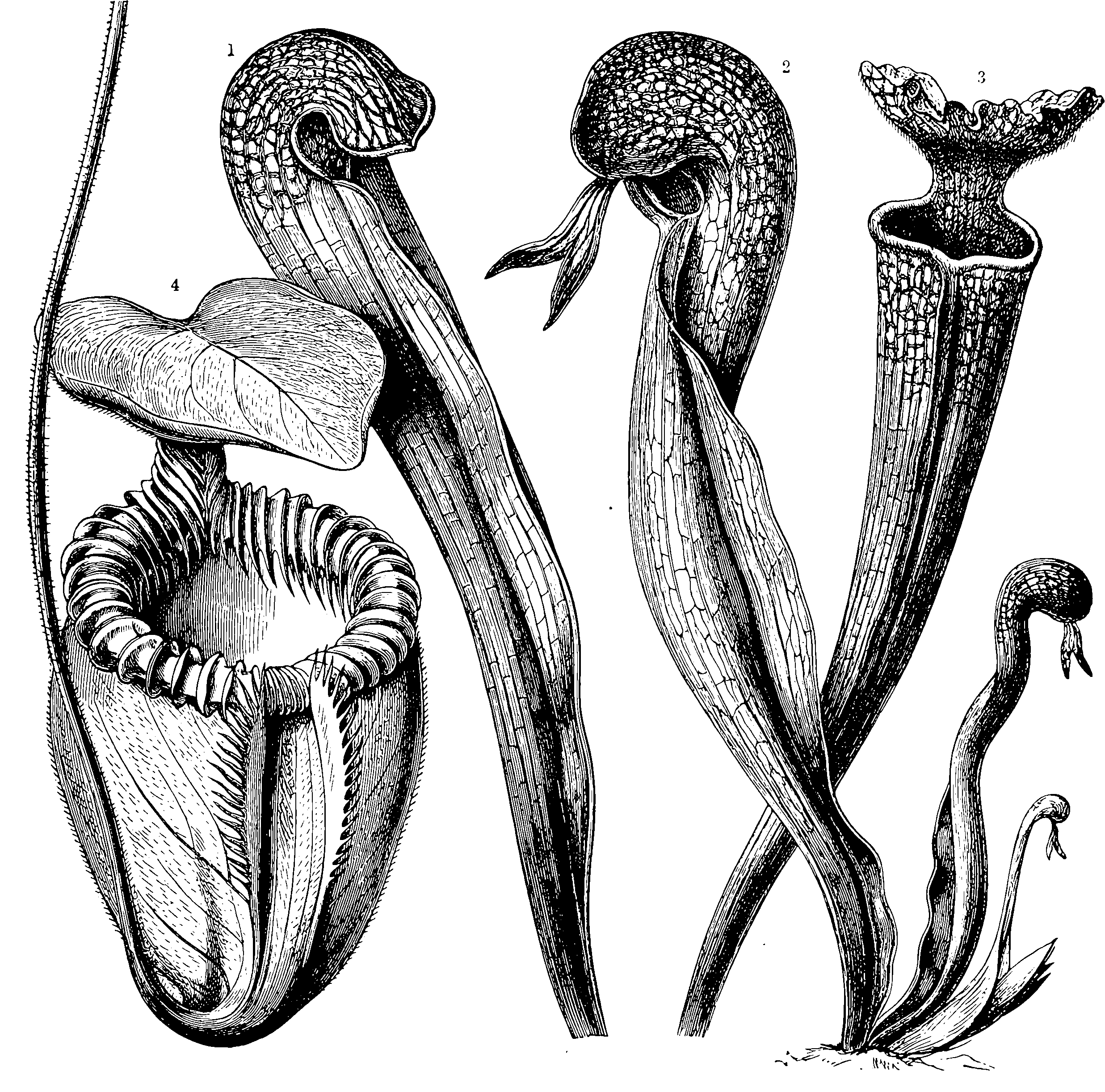# Generative flowPlaceholder. There are a lot of keywords in this new technique that sound intriguing, so here is a notebook to revisit if I ever have time.

Bengio et al. (2021):

Generative Flow Networks (GFlowNets) have been introduced as a method to sample a diverse set of candidates in an active learning context, with a training objective that makes them approximately sample in proportion to a given reward function. In this paper, we show a number of additional theoretical properties of GFlowNets. They can be used to estimate joint probability distributions and the corresponding marginal distributions where some variables are unspecified and, of particular interest, can represent distributions over composite objects like sets and graphs. GFlowNets amortize the work typically done by computationally expensive MCMC methods in a single but trained generative pass. They could also be used to estimate partition functions and free energies, conditional probabilities of supersets (supergraphs) given a subset (subgraph), as well as marginal distributions over all supersets (supergraphs) of a given set (graph). We introduce variations enabling the estimation of entropy and mutual information, sampling from a Pareto frontier, connections to reward-maximizing policies, and extensions to stochastic environments, continuous actions and modular energy functions.

### No comments yet. Why not leave one?

GitHub-flavored Markdown & a sane subset of HTML is supported.